NEET  >  Elasticity, Stress & Strain

# Elasticity, Stress & Strain - Notes | Study Physics Class 11 - NEET

 Table of contentsMechanical Properties of Solids1 Crore+ students have signed up on EduRev. Have you?

Mechanical Properties of Solids

Inter-molecular Force

In a solid, atoms and molecules are arranged in such a way that each molecule is acted upon by the forces due to the neighbouring molecules. These forces are known as inter molecular forces.

Elasticity

The property of the body to regain its original configuration (length, volume or shape) when the deforming forces are removed, is called elasticity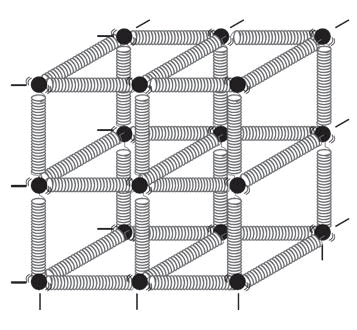Spring-ball model for the illustration of elastic behavior of solids

The change in the shape or size of a body when external forces act on it is determined by the forces between its atoms or molecules. These short range atomic forces are called elastic forces.

Perfectly elastic body

A body which regains its original configuration immediately and completely after the removal of deforming force from it is called perfectly elastic body. Quartz and phosphorus bronze are examples of nearly perfectly elastic bodies.

➤ Plasticity
The inability of a body to return to its original size and shape even on removal of the deforming force is called plasticity and such a body is called a plastic body.

Question for Elasticity, Stress & Strain
Try yourself:The ability of the material to deform without breaking is called:

Stress

Stress is defined as the ratio of the internal force F, produced when the substance is deformed, to the area A over which this force acts. In equilibrium, this force is equal in magnitude to the externally applied force. In other words,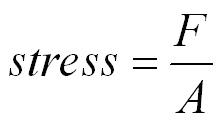The SI Unit of stress is newton per square meter (Nm-2).In CGS units, stress is measured in dyne cm-2. Dimensional formula of stress is ML-1T-2

Stress is of two types:
1. Normal stress: It is defined as the restoring force per unit area perpendicular to the surface of the body. Normal stress is of two types, tensile stress and compressive stress.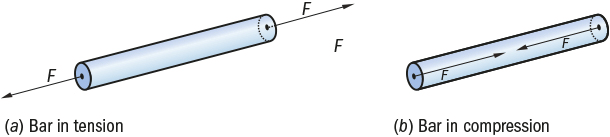Tensile and compressive stress

2. Tangential stress: When the elastic restoring force or deforming force acts parallel to the surface area, the stress is called tangential stress or shear stress.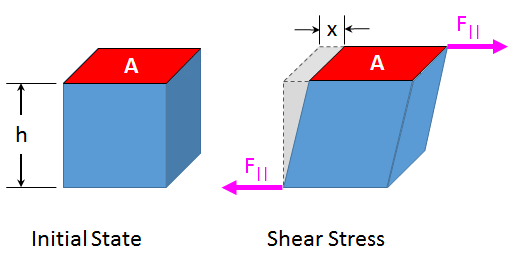Question for Elasticity, Stress & Strain
Try yourself:A 20kg load is suspended by a wire of cross section 0.4mm2. The stress produced in N/m2 is :

Strain

It is defined as the ratio of the change in size or shape to the original size or shape. It has no dimensions, it is just a number.

Strain is of three types:
1. Longitudinal strain: If the deforming force produces a change in length alone, the strain produced in the body is called longitudinal strain or tensile strain.
It is given as: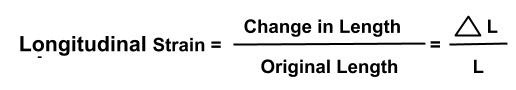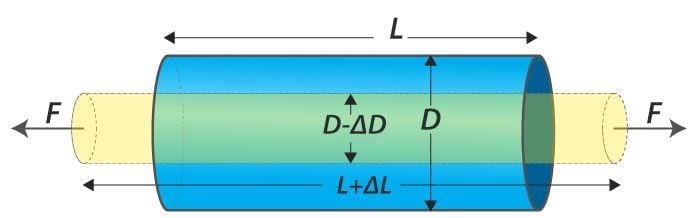Longitudinal strain

2. Volumetric strain: If the deforming force produces a change in volume alone, the strain produced in the body is called volumetric strain. It is given as: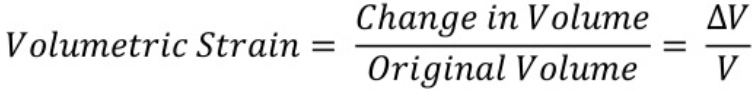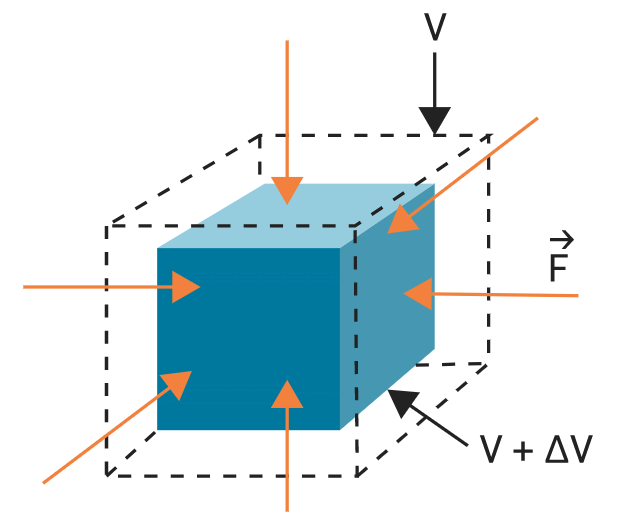Volumetric strain3. Shear strain: The angle tilt caused in the body due to tangential stress expressed is called shear strain. It is given as: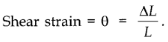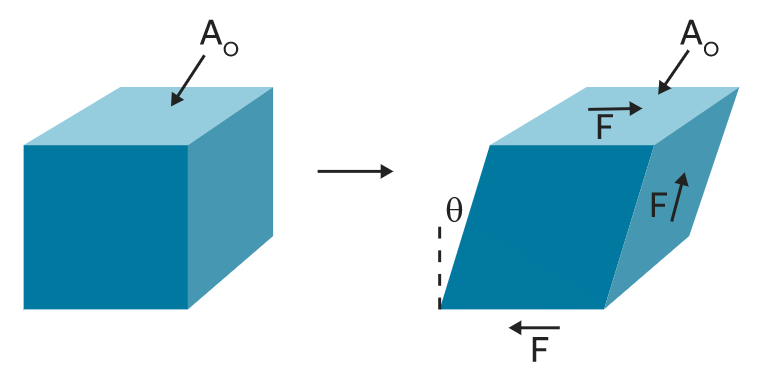Shear strain

The maximum stress to which the body can regain its original status on the removal of the deforming force is called elastic limit.

Question for Elasticity, Stress & Strain
Try yourself:Shearing strain is expressed by

Hooke’s Law

Hooke’s law states that, within elastic limits, the ratio of stress to the corresponding strain produced is a constant. This constant is called the modulus of elasticity. Thus,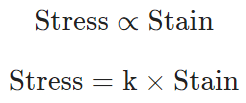Stress-Strain Curve

Stress-strain curves are useful to understand the tensile strength of a given material. The given figure shows a stress-strain curve of a given metal.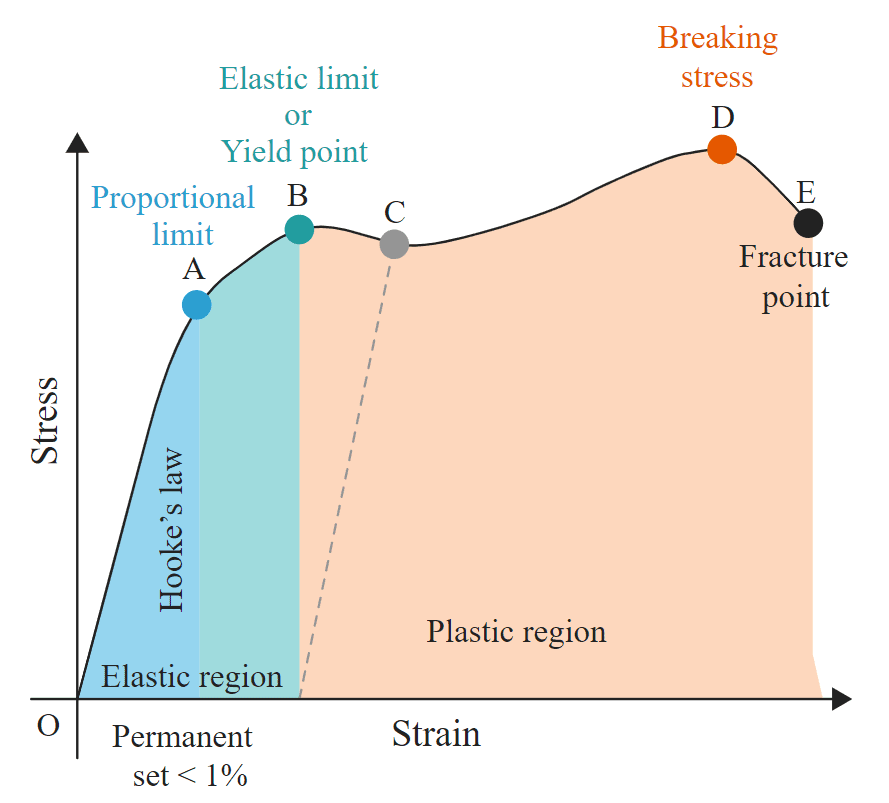Stress-strain curve

• The curve from O to A is linear. In this region Hooke’s Proportional limit law is obeyed.
• In the region from A to B stress and strain are not proportional. Still, the body regains its original dimension, once the load is removed.
• Point B in the curve is yield point or elastic limit and the corresponding stress is known as yield strength of the material.
• The curve beyond B shows the region of plastic deformation.
• The point D on the curve shows the tensile strength of the material. Beyond this point, additional strain leads to fracture, in the given material.
The document Elasticity, Stress & Strain - Notes | Study Physics Class 11 - NEET is a part of the NEET Course Physics Class 11.
All you need of NEET at this link: NEET

## Physics Class 11

127 videos|464 docs|210 tests

## Physics Class 11

127 videos|464 docs|210 tests

### How to Prepare for NEET

Read our guide to prepare for NEET which is created by Toppers & the best Teachers

Track your progress, build streaks, highlight & save important lessons and more!

,

,

,

,

,

,

,

,

,

,

,

,

,

,

,

,

,

,

,

,

,

,

,

,

;